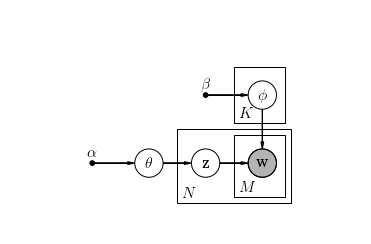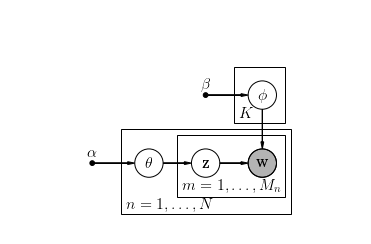topic-models slides

## Topic Models¶

### Simple Model: For Each Document One Topic¶

Naive bayes assumption: each document is drawn from a single topic (NOT mixture of topics) .

• $N$: number of documents in the collection.
• $M_n$: number of words in the $n$-th document.
• $V$: number of different words in the total collection
• $K$: number of predefined-topics (the number of topics is not automatically inferred)

Generative process:

1. Choose a word distribution $\phi_k$ for each topic $k$.
2. Choose a topic $z_i$ for each document $n$ from $\theta$. $z_i \in \{ 1,\dots, K \}$
3. For each of the word positions $m, n$, where $m \in \{ 1,\dots,M_n \}$, and $n \in \{ 1,\dots, N \}$
choose a word $w_{n,m} \,\sim\, \mathrm{Categorical}( \phi_{z_{i}})$.

#### Topic word distributions¶

• $\phi_k$: word distribution for the topics
$$\phi_k \sim Dirichlet(\beta)$$

with $1\leq k \leq K$ and each $\phi_k$ is a vector with $V$ elements.

Global (collection) topic distribution:

$$\theta \sim Dirichlet(\alpha)$$

Parameter for the probability that a document has topic $k$. $\theta$ is a vector with $K$ elements

$\alpha$ and $\beta$ are hyperparameters and fixed in our model.

$$z_n \sim Categorical(\theta)$$

with $1 \leq n \leq N$

In :
plot_naive_bayes_topic_model()In :
docs = ["football ball football ball ball ball football",
"money economy money money money economy economy",
"football ball football ball football football",
"economy economy money money",
"money economy computer economy",
"computer computer technology technology computer technology",
"technology computer technology",
"money economy economy money technology",
"computer technology computer technology"]

In :
from sklearn.feature_extraction.text import CountVectorizer
vectorizer = CountVectorizer()
v = vectorizer.fit_transform(docs)

In :
vectorizer.vocabulary_

Out:
{u'ball': 0,
u'computer': 1,
u'economy': 2,
u'football': 3,
u'money': 4,
u'technology': 5}
In :
v.toarray()

Out:
array([[4, 0, 0, 3, 0, 0],
[0, 0, 3, 0, 4, 0],
[2, 0, 0, 4, 0, 0],
[0, 0, 2, 0, 2, 0],
[0, 1, 2, 0, 1, 0],
[0, 3, 0, 0, 0, 3],
[0, 1, 0, 0, 0, 2],
[0, 0, 2, 0, 2, 1],
[0, 2, 0, 0, 0, 2]])
In :
def to_one_word_array(v):
docs = list()
for w in v.toarray():
words = list()
for d, n in enumerate(w):
for j in range(n):
words.append(d)
#print d
docs.append(words)
return docs

In :
documents = to_one_word_array(v)

In :
documents

Out:
[[0, 0, 0, 0, 3, 3, 3],
[2, 2, 2, 4, 4, 4, 4],
[0, 0, 3, 3, 3, 3],
[2, 2, 4, 4],
[1, 2, 2, 4],
[1, 1, 1, 5, 5, 5],
[1, 5, 5],
[2, 2, 4, 4, 5],
[1, 1, 5, 5]]
In :
# number of topics
K = 3

In :
# number of words
V = len(vectorizer.vocabulary_)
V

Out:
6
In :
#number of documents
N = len(docs)
N

Out:
9
In :
#array containing the information about doc length in our collection
m_n = [m.sum() for m in v]
m_n

Out:
[7, 7, 6, 4, 4, 6, 3, 5, 4]
In :
# Hyperparameter: alpha, beta

# D: number of documents
# K: number of predefined topics
alpha = np.ones((K,))

#V: number of vocabulary terms
beta = np.ones((V,))

In :
# word distribution for each topic k
# note beta has dim V so also phi.
phi_ = [pymc.Dirichlet("pphi_%i" % k, theta=beta) for k in range(K)]
phi  = [pymc.CompletedDirichlet("phi_%i" % k, phi_[k]) for k in range(K)]

# each document belongs exactly to a topic z
theta = pymc.Dirichlet("theta", theta=alpha)
z = [pymc.Categorical("z_%i"%n, p=theta) for n in range(N)]

# version with multinominal:
#words_in_docs = [pymc.Multinomial("words_in_doc_%i"%(d,), value=v[d].toarray(), observed=True,
#                   n=n, p=pymc.Lambda("phi_%i"%(d),  lambda z=z[d], phi=phi : phi[z]))
#                    for d, n in enumerate(Nd)]

w = [pymc.Categorical("w_%i_%i" % (n, m),
p = pymc.Lambda("phi_z_%i_%i" % (n, m),
lambda z=z[n], phi=phi : phi[z]),
value=documents[n][m],
observed=True)
for n in range(N) for m in range(m_n[n])]

In :
documents

Out:
4
In :
mcmc = pymc.MCMC([phi_, phi, theta, z, w])
mcmc.sample(10000, burn=5000)

 [-----------------100%-----------------] 10000 of 10000 complete in 43.7 sec
In :
vectorizer.vocabulary_

Out:
{u'ball': 0,
u'computer': 1,
u'economy': 2,
u'football': 3,
u'money': 4,
u'technology': 5}
In :
inv_voc = {v: k for k, v in vectorizer.vocabulary_.items()}
inv_voc

Out:
{0: u'ball',
1: u'computer',
2: u'economy',
3: u'football',
4: u'money',
5: u'technology'}
In :
for k in range(K):
print "topic %i"%k
for i, j in enumerate(mcmc.trace('phi_%i'%k)[-100:-1].mean(axis=0)):
print "\t", inv_voc[i], ":", j
print

topic 0
ball : 0.0363931429669
computer : 0.256455662602
economy : 0.0402787781787
football : 0.0705131792063
money : 0.109059925401
technology : 0.487299311644

topic 1
ball : 0.0149182117232
computer : 0.0822085487173
economy : 0.385940319504
football : 0.0417620171837
money : 0.4026711362
technology : 0.0724997666716

topic 2
ball : 0.27412201123
computer : 0.0610294392576
economy : 0.133883284225
football : 0.421780433754
money : 0.0507701509037
technology : 0.0584146806298


In :
for n in range(N):
print(mcmc.trace('z_%i'%n)[-1])

2
1
2
1
1
0
0
1
0

In :
np.random.rand()

Out:
0.346432025127938

### LDA - Latent Dirichlet Allocation¶

#### Smoothed LDA¶

The generative process is as follows. Documents are represented as random mixtures over latent topics, where each topic is characterized by a distribution over words. LDA assumes the following generative process for a corpus $D$ consisting of $N$ documents each of length $m_n$:

1. Choose $m \sim \mathrm{Poission}(\zeta)$, the number of terms in the document.
2. Choose a word distribution $\phi_k \sim \, \mathrm{Dir}(\beta)$ for each topic $k$
3. Choose $\theta_n \, \sim \, \mathrm{Dir}(\alpha)$, where $n \in \{ 1,\dots, N \}$
1. $\theta_n$ is the topic distribution for document $n$
2. and $\mathrm{Dir}(\alpha)$ is the Dirichlet distribution for parameter $\alpha$
4. For each of the word positions $n, m$, where $m \in \{ 1,\dots,M_i \}$, and $n \in \{ 1,\dots, N \}$
a. Choose a topic $z_{n,m} \,\sim\, \mathrm{Categorical}(\theta_n).$
b. Choose a word $w_{n,m} \,\sim\, \mathrm{Categorical}( \beta_{z_{n,m}})$.
• $\alpha$: parameter of Dirichlet prior on per-document topic distribution ($m$-Vector)
• $\beta$: parameter of Dirichlet prior on per-word topic distribution ($k \times V$-Matrix)
• $\theta_n$ topic distribution for document $n$
• $z_{n, m}$ topic for the $m$th word in document $n$
• $w_{n,m}$ specific word $m$ in document $n$

• $V$ number of words in the vocabulary

Exercise: Write down the graph factorization as formula: $$p(\theta, \bf z, \bf w \mid \alpha, \beta) = ?$$

In :
plot_smoothed_lda()In :
# Hyperparameter: alpha, beta

# D: number of documents
# K: number of predefined topics
alpha = np.ones((K,))

#V: number of vocabulary terms
beta = np.ones((V,))

In :
D=N
Nd = m_n
# word distribution for each topic k
phi =[pymc.CompletedDirichlet("phi_%i" % k, phi_[k]) for k in range(K)]

# each document has a topic distribution
theta = [pymc.Dirichlet("theta_%i"%d, theta=alpha) for d in range(D)]

z = [pymc.Categorical("z_%i" % d,  p = theta[d],
size = Nd[d],
value = np.random.randint(K, size=Nd[d]))
for d in range(D)]

#word generated from phi, given a topic z
w = [pymc.Categorical("w_%i_%i" % (d,i),
p = pymc.Lambda("phi_z_%i_%i" % (d,i),
lambda z=z[d][i], phi=phi : phi[z]),
value=documents[d][i],
observed=True)
for d in range(D) for i in range(Nd[d])]


In :
mcmc = pymc.MCMC([phi_, phi, theta, z, w])
mcmc.sample(10000, burn=8000)

 [-----------------100%-----------------] 10000 of 10000 complete in 219.4 sec
In :
#show the topic assignment for each document
for d in range(D):
#print mcmc.trace('z_%i'%d)[-1]
print np.bincount(mcmc.trace('z_%i'%d)[-1], minlength=3)

# number of choosen topics for the documents (sums up to the number of terms)
# only for the last sample of the MCMC trace

[7 0 0]
[0 7 0]
[6 0 0]
[0 4 0]
[0 2 2]
[0 0 6]
[0 0 3]
[0 4 1]
[0 2 2]

In :
for k in range(K):
print "topic %i"%k
for i, j in enumerate(mcmc.trace('phi_%i'%k)[-100:-1].mean(axis=0)):
print "\t", inv_voc[i], ":", j
print

topic 0
ball : 0.494302656478
computer : 0.00330601256377
economy : 0.0113991691287
football : 0.418033392202
money : 0.039234887214
technology : 0.0337238824139

topic 1
ball : 0.0144646150808
computer : 0.0642180078031
economy : 0.494408160655
football : 0.0146421743477
money : 0.352925984262
technology : 0.0593410578516

topic 2
ball : 0.00538960882665
computer : 0.411470519517
economy : 0.0136952757781
football : 0.048737756214
money : 0.0305771090712
technology : 0.490129730593